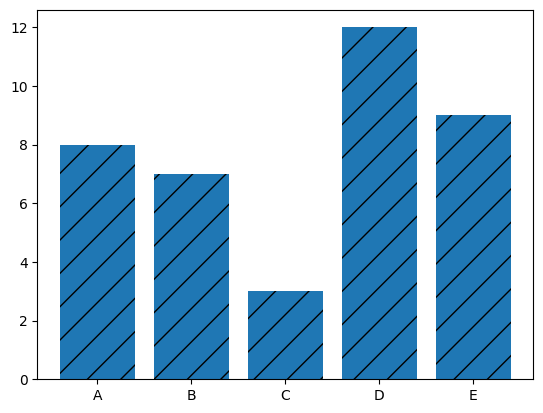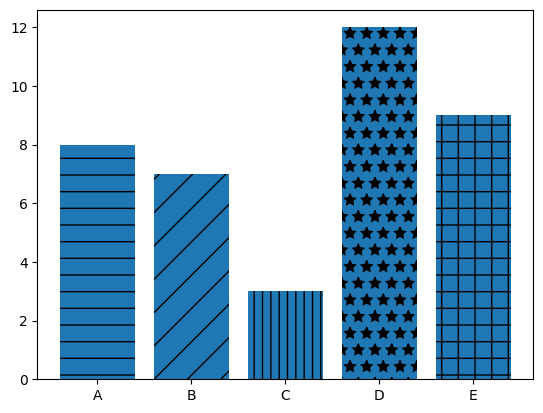## Libraries

First, you need to import the following libraries:

• matplotlib for creating the chart and customize it
• pandas for storing the data used
``````import matplotlib.pyplot as plt
import pandas as pd``````

## Dataset

In this post, we'll use a very simple dataset, that you can create as follow

``````height = [8, 7, 3, 12, 9]
bars = ('A', 'B', 'C', 'D', 'E')

df = pd.DataFrame({'height': height,
'bars': bars})``````

## Define the Hatch

This example provides a reproducible code in which you can change the texture of the bars using the `set_hatch()` function of matplotlib.

It must be in the following list: `'/'`, `'\\'`, `'|'`, `'-'`, `'+'`, `'x'`, `'o'`, `'O'`, `'.'`, `'*'`

``````# Create a figure and axis
fig, ax = plt.subplots()

# Create bars
ax.bar(df['bars'], df['height'],
hatch='/')

# Show the graphic
plt.show()``````## Multiple hatchs

If you want to have a different hatch for each bar, we need to make some modifications in the initial code:

• define a list of hatches (with the same length as the number of bars)
• iterate over each bar and set the wanted hatch
``````# Create a figure and axis
fig, ax = plt.subplots()

# Create bars
bars = ax.bar(df['bars'], df['height'])

# Define some hatches
hatches = ['-', '/', '||', '*', '+']
for bar, hatch in zip(bars, hatches):
bar.set_hatch(hatch)

# Show the graphic
plt.show()``````## Going further

This post explains how to change texture of a barplot with matplotlib.

You might want to check how to custom width of bars and how to change colors of the bars.

## Contact & Edit

👋 This document is a work by Yan Holtz. You can contribute on github, send me a feedback on twitter or subscribe to the newsletter to know when new examples are published! 🔥